Function Repository Resource:

# SafePrimeQ

Determine whether a number is a safe prime

Contributed by: Arnoud Buzing
 ResourceFunction["SafePrimeQ"][p] gives True if p is a safe prime and False otherwise.

## Details and Options

A safe prime is a prime number of the form 2q+1, where q is also a prime. Here, q is referred to as a Sophie Germain prime.

## Examples

### Basic Examples (3)

23 is a safe prime, because both 23=2·11+1 and 11 are primes:

 In:=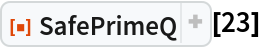Out=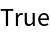29 is not a safe prime, because 29=2·14+1 but 14 is not prime:

 In:=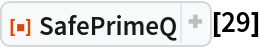Out=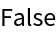Even though 15=2·7+1 and 7 is a prime number, 15 is not a safe prime because it is not prime itself:

 In:=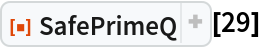Out=Arnoud Buzing

## Version History

• 1.0.0 – 29 April 2020